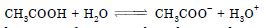# NCERT Solutions for Class 12 Chemistry Chapter 3 - Electrochemistry

NCERT Solutions plays an important role in the student’s journey of exam preparation. Professional teachers design the solutions in a way that students find no issue in grasping the concepts included in the latest syllabus. The NCERT Solutions for Class 12 at Aasoka has made sure to follow the latest CBSE syllabus so as not to miss out on anything important. These solutions will answer all the queries of students of Class 12 regarding the topic “Electrochemistry”.

The Class 12 Chemistry chapter “Electrochemistry” covers topics like electrolysis, Nernst equation, the relation between Gibbs energy change and EMF of a cell, specific and molar conductivity, Kohlrausch’s law, the conductance of electrolytic solutions, standard electrode potential, variations of conductivity with concentration, electrolytic cells, electrochemical cells, redox reactions, etc.

##### Question 1: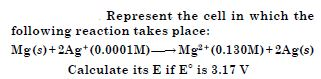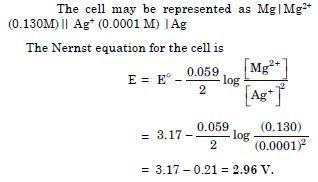##### Question 2:

Calculate the equilibrium constant for the reaction at 298 K.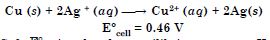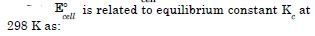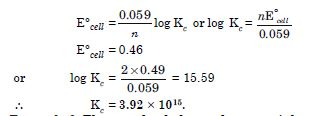##### Question 3:

The standard electrode potential of Daniell cell is 1.1 V. Calculate the standard Gibbs energy change for the reaction: Zn (s) + Cu2+ (aq) $\to$ Zn2+ (aq) + Cu(s)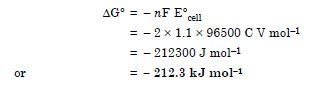##### Question 4:

Resistance of a conductivity cell filled with 0.1 M KCl solution is 100 $\mathrm{\Omega }$. If the resistance of the same cell when filled with 0.02 M KCl solution is 520 $\mathrm{\Omega }$, calculate the conductivity and molar conductivity of 0.02 M KCl solution. (The conductivity of 0.1 M KCl solution is 1.29 S m–1)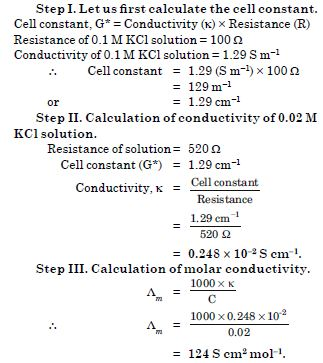##### Question 5:

The electrical resistance of a column of 0.05 M NaOH solution of diameter 1 cm and length 50 cm is 5.55 × 103 ohm. Calculate its resistivity, conductivity and molar conductivity.

R = 5.55 × 103 ohm, C = 0.05 M, l = 50 cm, diameter = 1 cm, radius = 0.5 cm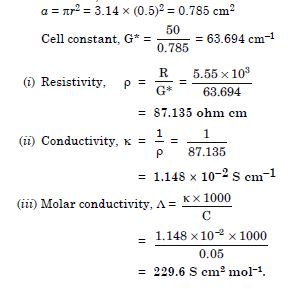##### Question 6:

The molar conductivity of KCl solution at different concentration at 298 K are given below :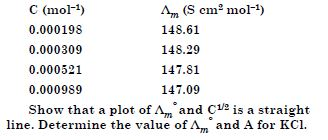Taking the square root of concentration, we have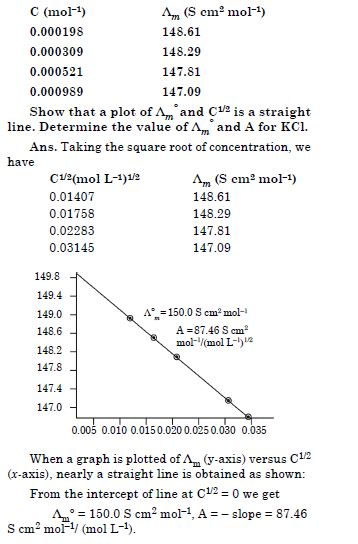##### Question 7: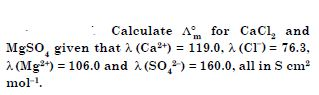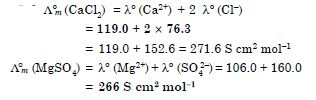##### Question 8: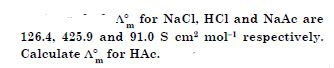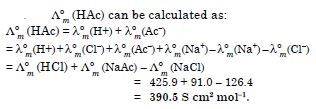##### Question 9:

The conductivity of 0.001028
mol L–1 acetic acid is 4.95 × 10–5 S cm–1. Calculate its dissociation constant if $\mathrm{\Lambda }$ (CH3COOH) is 390.5 S cm2 mol–1.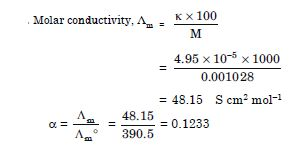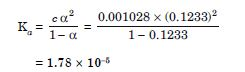##### Question 10:

A solution of CuSO4 is electrolysed for 10 minutes with a current of 1.5 amperes. What is the mass of copper deposited at the cathode ?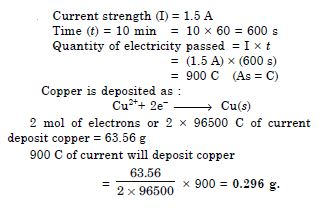##### Question 11:

How would you determine the standard electrode potential of the system Mg2+|Mg ?

The standard electrode potential of Mg2+|Mg system can be measured by connecting the Mg electrode immersed in 1M Mg2+ solution with S.H.E. This may be represented as :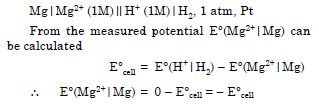##### Question 12:

Can you store copper sulphate solution in a zinc pot ?

No, because zinc is more reactive than copper and it will displace copper from copper sulphate solution as :

Zn(s) + CuSO4 (aq) $\to$ ZnSO4(aq) + Cu(s)
As a result, zinc will gradually pass into the solution and copper will be precipitated.

##### Question 13:

Consult the table of standard electrode potentials and suggest three substances which can oxidize ferrous ions under suitable conditions.

The electrode potential of Fe3+|Fe2+ is 0.78 V. The substances having electrode potential more than 0.78 V will be reduced and hence act as oxidising agent. For example,

1. acidified KMnO4
2. acidified Cr2O72–
3. acidified H2O2.
##### Question 14:

Calculte the potential of hydrogen electrode in contact with a solution whose pH is 10.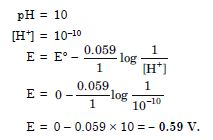##### Question 15:

Calculate the e.m.f of the cell in which the following reaction takes place :
Ni(s) + 2Ag+ (0.002 M) $\to$ Ni2+ (0.160 M) + 2Ag
Given that E$°$cell = 1.05 V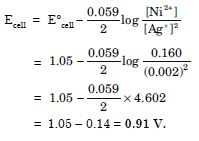##### Question 16:

The cell in which the following reaction occurs :
2Fe3+(aq) + 2I(aq) $\to$ 2Fe2+(aq) + I2(s) has E$°$cell = 0.236 V at 298 K. Calculate the standard Gibbs energy and the equilibrium constant of the cell reaction.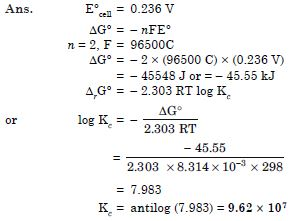##### Question 17:

Why does the conductivity of a solution decrease with dilution ?

Conductivity of an electrolyte solution decreases with dilution because the number of ions per unit volume furnished by an electrolyte decreases with dilution.

##### Question 18:

Suggest a way to determine $\mathrm{\Lambda }$$°$m value of water.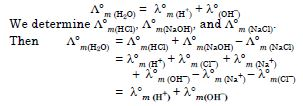##### Question 19:

The molar conductivity of 0.025 mol L–1 methanoic acid is 46.1 S cm2 mol–1. Calculate its degree of dissociation and dissociation constant. Given $\mathrm{\lambda }$$°$ (H+) = 349.6 S cm2 mol–1 and $\mathrm{\lambda }$$°$ (HCOO–­) = 54.6 S cm2 mol–1.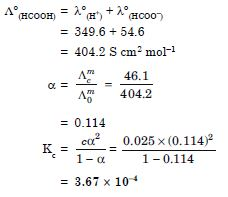##### Question 20:

If a current of 0.5 ampere flows through a metallic wire for 2 hours, then how many electrons would flow through the wire ?

Coulombs of electrons = Current × time
= 0.5 A × 2 × 60 × 60s
= 3600 C
Now, the current of 1F (96500 C) is equivalent to 1 mole i.e., 6.022 × 1023 electrons.
$\therefore$ 3600 C of current is equivalent to flow of electrons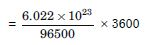= 2.246 × 1022 electrons.

##### Question 21:

Suggest a list of metals that are extracted electrolytically.

Sodium, potassium, calcium, magnesium, aluminium, etc.

##### Question 22:

Consider the reaction :
Cr2O72– + 14H+ + 6e $\to$ 2Cr3+ + 8H2O What is the quantity of electricity in coulombs needed to reduce 1 mol of Cr2O72– ?

6 mol of electrons are required to reduce 1 mol of Cr2O72–
Now, 1 mol of electrons = 96500 C
6 mol of electrons = 96500 × 6
= 5.79 × 105 C

##### Question 23:

Write the chemistry of recharging of the lead storage battery, highlighting all the materials that are involved during discharging.

A lead storage battery consists of anode of lead, cathode of a grid of lead packed with lead dioxide (PbO2) and 38% H2SO4 solution as electrolyte. The following reactions occur when the battery is in use :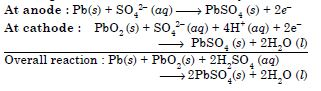On charging the battery, the reverse reaction takes place i.e., PbSO4 deposited on the electrodes is converted back into lead and PbO2 and H2SO4 are regenerated.

##### Question 24:

Suggest two materials other than hydrogen that can be used as fuels in fuel cells.

(i) Methane (ii) Ethane Recently zinc metal has been used in place of hydrogen gas in USA to develop a source of power for automobiles.

##### Question 25:

Explain how rusting of iron is envisaged as setting up of an electrochemical cell.

Water layer present on the surface of iron (particularly during rainy season) dissolves acidic oxides present in air such as CO2, SO2, etc. to form acids which dissociate to give H+ ions: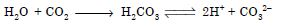In the presence of H+ ions, iron starts losing electrons at a particular spot of iron object to form ferrous ions. Therefore, oxidation takes place and that spot behaves as anode.
Fe(s) $\to$ Fe2+ (aq) + 2e
The electrons released at anodic spot move through the metal to reach another spot where H+ ions and the dissolved oxygen take up these electrons and reduction takes place. Hence, this spot behaves as cathode
O2(g) + 4H+ (aq) + 4e $\to$ 2H2O(l)
The overall reaction is :
2Fe(s) + O2(g) + 4H+(aq) $\to$ 2Fe2+(aq) + 2H2O(l)
Thus, an electrochemical cell sets up on the surface. Ferrous ions are further oxidised by atmospheric oxygen to ferric ions which combine with water molecules to form hydrated ferric oxide, Fe2O3. xH2O, which is rust.

##### Question 26:

Arrange the following metals in the order in which they displace each other from the solution of their salts
Al, Cu, Fe, Mg and Zn.

Mg, Al, Zn, Fe, Cu.

##### Question 27:

Given the standard electrode potentials,
K+/K = – 2.93 V, Ag+/Ag = 0.80 V
Hg2+/Hg = 0.79 V
Mg2+/Mg = – 2.37 V, Cr3+/Cr = – 0.74V
Arrange these metals in their increasing order of reducing power.

Ag+/Ag, Hg2+/Hg, Cr3+/Cr, Mg2+/Mg, K+/K

##### Question 28:

Depict the galvanic cell in which the reaction
Zn(s) + 2Ag+(aq) $\to$ Zn2+(aq) + 2 Ag(s)
takes place. Further show :

1. Which of the electrode is negatively charged ?
2. The carriers of the current in the cell.
3. Individual reaction at each electrode.

The cell may be represented as :
Zn(s)|Zn2+(aq)||Ag+(aq)|Ag(s)

1. Zinc electrode (anode)
2. Current will flow from silver to zinc in the external circuit.
3. At anode : Zn(s) $\to$ Zn2+ + 2e At cathode : Ag+(aq) + e $\to$ Ag(s)
##### Question 29:

Calculate the standard cell potentials of galvanic cells in which of the following reactions take place :

1. 2Cr(s) + 3Cd2+(aq) $\to$ 2Cr3+(aq) + 3Cd(s)
2. Fe2+ (aq) + Ag+(aq) $\to$ Fe3+(aq) + Ag(s)
Calculate the $∆$rG$°$ and equilibrium constant of the reactions.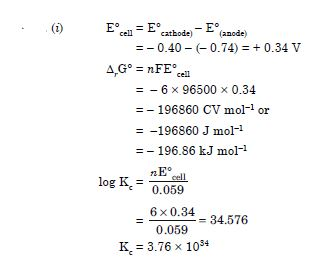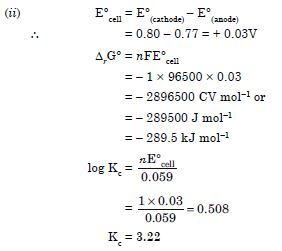##### Question 30:

Write the Nernst equation and calculate emf of the following cells at 298 K:

1. Mg(s)| Mg2+(0.001M)$\parallel$ Cu2+(0.0001M)|Cu(s)
2. Fe(s)| Fe2+(0.001M)$\parallel$ H+(1M)| H2(g)(1bar)| Pt(s)
3. Sn(s)| Sn2+(0.050M)$\parallel$ H+(0.020M)| H2(g) (1bar)| Pt(s)
4. Pt(s)|Br2(l)| Br (0.010M)$\parallel$ H+(0.030M)| H2(g) (1bar) | Pt(s)
1. (i) The electrode reactions and cell reactions are: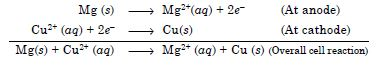Since the reaction involves 2 moles of electrons and therefore, n = 2 and the Nernst equation for the cell at 298 K is :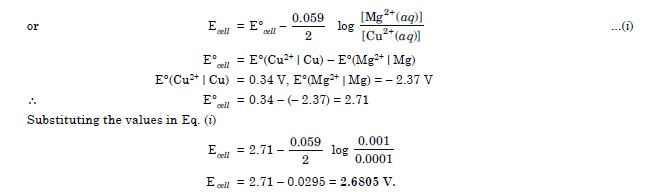2. Fe(s) | Fe2+(0.001 M) || H+ (1M) | H2 (1 atm) | Pt
The electrode reactions and overall cell reactions are :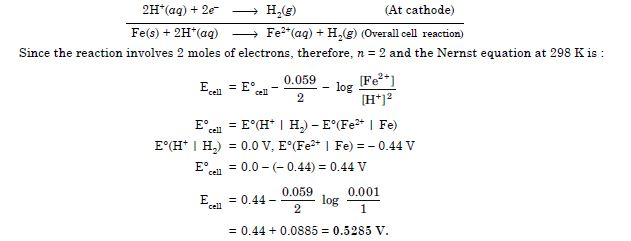3. The cell is :
Sn(s) | Sn2+(0.050M) || H+(0.020M) | H2(1 atm) | Pt
The electrode reactions and cell reactions are :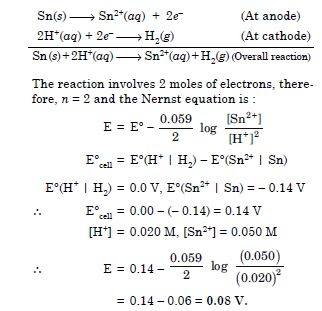4. Pt(s)|Br2(l)|Br–(0.010M)||H+(0.030M)|H2(g) (1bar)|Pt(s)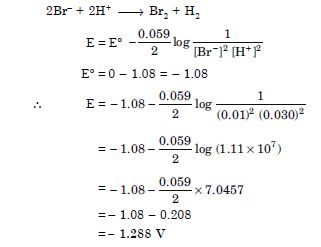The given reaction is not feasible. Thus, oxidation will occur at the hydrogen electrode and reduction on the Br2 electrode and E$°$cell = 1.288 V.
##### Question 31:

In the button cells widely used in watches and other devices the following reaction takes place :
Zn(s) + Ag2O(s) + H2O(l) $\to$ Zn2+(aq) + 2Ag(s) + 2OH(aq)
Determine $∆$rG$°$ and E$°$ for the reaction. (Given Eº(Zn2+|Zn) = –0.76V, E$°$ (Ag2O|Ag)
= –0.344V)

(a) Zn is oxidised and Ag2O is reduced.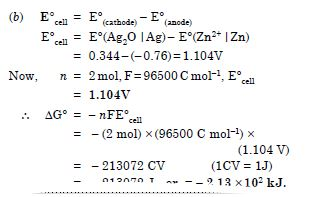##### Question 32:

Define conductivity and molar conductivity for the solution of an electrolyte. Discuss their variation with concentration.

Conductivity is the conductance between opposite faces of 1 centimetre cube of a conductor. It is denoted by κ (kappa) and has the units ohm–1 cm–1.

Molar conductivity is the conducting power of all the ions produced by dissolving one gram mole of an electrolyte in solution. It is expressed as Am and has the units ohm–1 cm2 mol–1.

Molar conductivity (Am) and specific conductivity (κ) are related as :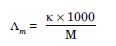where M is the molarity of the solution.
Variation of molar conductivity with dilution
The molar conductance of an electrolytic solution decreases with increase in concentration. For weak electrolytes, molar conductivity increases sharply with dilution. On the other hand, for strong electrolytes, molar conductivity increases slowly with dilution.
For weak electrolytes, the increase in molar conductivity is due to increase in degree of ionisation with dilution. For strong electrolytes, the increase in molar conductance with dilution is because of decrease in interactions between ions with dilution. The decrease in molar conductivity for weak electrolyte (CH3COOH) and strong electrolyte (KCl) is shown below :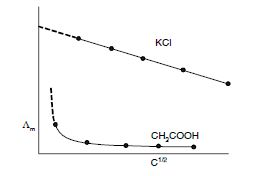##### Question 33:

The conductivity of 0.20 M solution of KCl at 298K is 0.0248 S cm–1. Calculate its molar conductivity.

Molar conductivity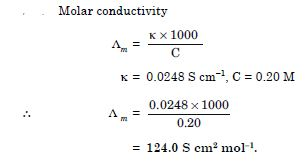##### Question 34:

The resistance of a conductivity cell containing 0.001M KCl solution at 298 K is 1500$\mathrm{\Omega }$. What is the cell constant if conductivity of 0.001 M KCl solution at 298 K is 0.146 × 10–3 S cm–1?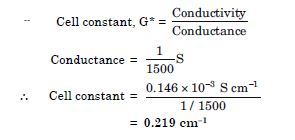##### Question 35:

The conductivity of sodium chloride at 298 K has been determined at different concentrations and the results are given below :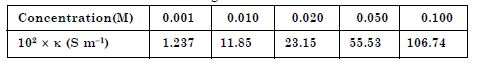Calculate $\mathrm{\Lambda }$m for all concentrations and draw a plot between $\mathrm{\Lambda }$m and C1/2. Find the value of $\mathrm{\Lambda }$$°$m.

$\mathrm{\Lambda }$m at different concentrations may be calculated as :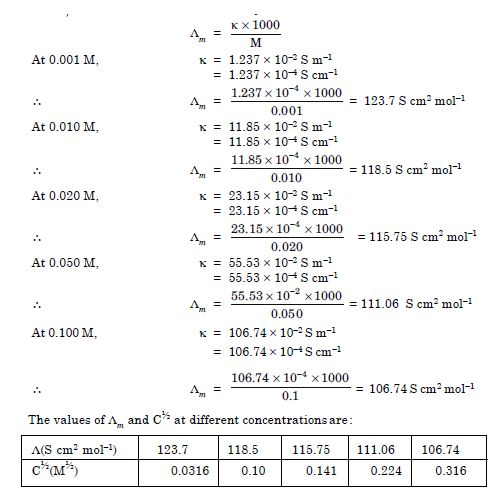Plot of $\mathrm{\Lambda }$m and C½ is given below: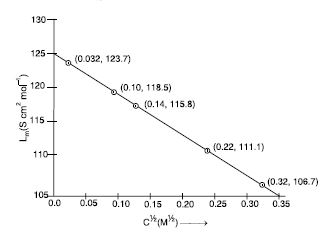The extrapolation of the straight line to zero concentration (intercept) gives the value of $\mathrm{\Lambda }$$°$m = 124.5 S cm2 mol–1.

##### Question 36:

Conductivity of 0.00241M acetic acid is 7.896 × 10–5S cm–1. Calculate its molar conductivity and if $\mathrm{\Lambda }$$°$m for acetic acid is
390.5 S cm2 mol–1, what is its dissociation constant ?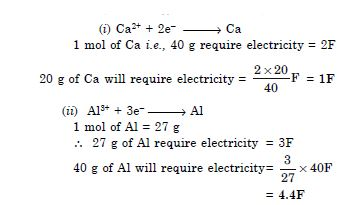##### Question 37:

How much electricity is required in coulomb for the oxidation of

1. 1 mol of H2O to O2 ?
2. 1 mol of FeO to Fe2O3 ?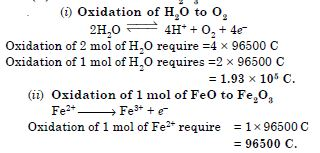##### Question 38:

A solution of Ni(NO3)2 is electrolysed between platinum electrodes using a current of 5 amperes for 20 minutes. What mass of Ni is deposited at the cathode?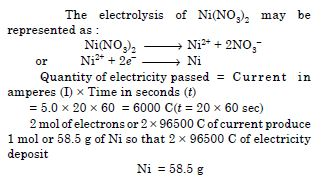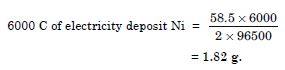##### Question 39:

Three electrolytic cells A, B, C containing solution of ZnSO4, AgNO3 and CuSO4, respectively are connected in series. A steady current of 1.5 amperes was passed through them until 1.45 g of silver deposited at the cathode of cell B. How long did the current flow? What mass of copper and of zinc were deposited?

Cell B contains AgNO3 and reaction may be represented as :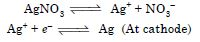According to the equation,
1 mol or 108 g of silver is deposited by 96500 C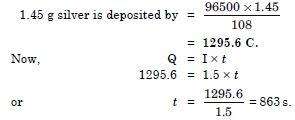The weights of copper and zinc can be calculated by using Faraday’s second law of electrolysis.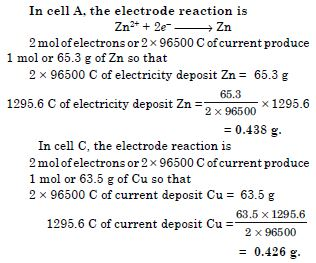##### Question 40:

Predict if the reaction between the following is feasible :

1. Fe3+ (aq) and I (aq)
2. Ag+ (aq) and Cu (s)
3. Fe3+ (aq) and Br (aq)
4. Ag(s) and Fe3+(aq)
5. Br2 (aq) and Fe2+ (aq)

Given standard electrode potentials :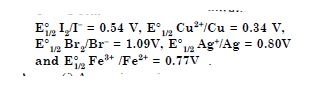(i) A reaction is feasible if EMF of the cell is positive.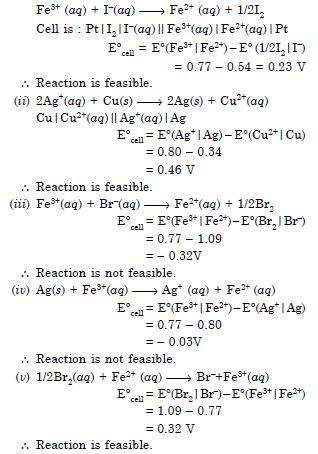##### Question 41:

Predict the products of electrolysis in each of the following :

1. An aqueous solution of AgNO3 with silver electrode.
2. An aqueous solution of AgNO3 with platinum electrodes.
3. A dilute solution of H2SO4 with platinum electrodes.
4. An aqueous solution of CuCl2 with platinum electrodes.

Electrolysis of aqueous solution of AgNO3 using silver electrodes :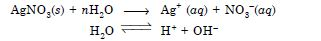At cathode : Ag+ ions have lower discharge potential than H+ ions. Hence Ag+ ions will be deposited as silver (in preference to H+ ions).
At anode : Since silver electrode is attacked by NO3 ions, Ag anode will dissolve to form Ag+ ions in the solution.
Ag  ¾→ Ag+ + e

(ii) Electrolysis of aqueous solution of AgNO3 using platinum electrodes
At cathode : Same as above.
At anode : Since silver is not attacked, out of OH– and NO3– ions, OH– ions have lower discharge potential and hence OH– ions will be discharged in preference to NO3–. The OH– will decompose to give O2.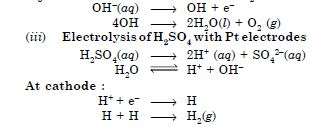At anode :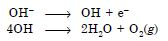(iv) Electrolysis of aqueous solution of CuCl2 with platinum electrodes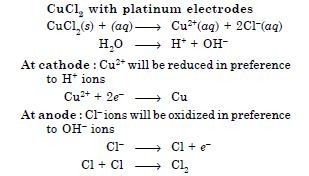##### Question 42:

Can absolute electrode potential of an electrode be measured?

No, absolute electrode potential of an electrode cannot be measured.

##### Question 43:

Can E$°$cell or DrG$°$ for a cell reaction ever be equal to zero?

No, $∆$G$°$ = nFE$°$, if nFE$°$ is zero, cell reaction will not occur.

##### Question 44:

Under what condition is ECell = 0 or $∆$rG = 0?

When the cell reaction reaches equilibrium, Ecell = 0, so that $∆$G is also zero.

##### Question 45: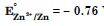V mean?

It means that zinc is more reactive than hydrogen. When zinc electrode is connected to SHE, zinc will get oxidised and H+ will get reduced.

##### Question 46:

Aqueous copper sulphate solution and aqueous silver nitrate solution are electrolysed by 1 Ampere current for 10 minutes in separate electrolytic cells. Will the mass of copper and silver deposited on the cathode be same or different? Explain your answer.

Mass of copper and silver deposited on cathode will be different. It depends upon equivalent mass.

##### Question 47:

Depict the galvanic cell in which the cell reaction is Cu + 2Ag+ $\to$ 2Ag + Cu2+

Cu|Cu2+ (aq, 1M) $\parallel$ Ag+ (aq, 1M) |Ag.

##### Question 48:

Value of standard electrode potential for the oxidation of Cl ions is more positive than that of water, even then in the electrolysis of aqueous sodium chloride, why is Cl oxidised at anode instead of water?

Under the conditions of electrolysis of aqueous sodium chloride, oxidation of water at anode requires over potential and therefore, Cl is oxidised instead of water.

##### Question 49:

What is electrode potential?

The electrical potential difference set up between the metal and its solution is called electrode potential.

##### Question 50:

Consider the following diagram in which an electrochemical cell is coupled to an electrolytic cell. What will be the polarity of electrodes ‘A’ and ‘B’ in the electrolytic cell?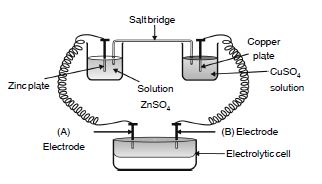‘A’ will have –ve polarity and ‘B’ will have +ve polarity.

##### Question 51:

Why is alternating current used for measuring resistance of an electrolytic solution?

The alternating current is used to prevent electrolysis so that the concentration of ion in the solution remains constant.

##### Question 52:

A galvanic cell has electrical potential of 1.1V. If an opposing potential of 1.1V is applied to this cell, what will happen to the cell reaction and current flowing through the cell?

When the opposing potential becomes equal to electrical potential, the cell reaction stops and no current flows through the cell. Thus, there is no chemical reaction.

##### Question 53:

How will the pH of brine (aq. NaCl solution) be affected when it is electrolysed?

Since NaOH is formed during electrolysis, pH of the brine solution will increase

##### Question 54:

Unlike dry cell, the mercury cell has a constant cell potential throughout its life. Why?

Beacuse ions are not involved in the overall cell reaction of mercury cells.

##### Question 55:

Solutions of two electrolytes ‘A’ and ‘B’ are diluted. The $\mathrm{\Lambda }$m of ‘B’ increases 1.5 times while that of A increases 25 times. Which of the two is a strong electrolyte? Justify your answer.

Electrolyte B is strong because on dilution $\mathrm{\Lambda }$m increases only small.

##### Question 56:

When acidulated water (dil.H2SO4 solution) is electrolysed, will the pH of the solution be affected? Justify your answer.

pH of the solution remains same because [H+] remains constant.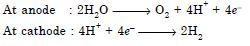##### Question 57:

In an aqueous solution how does specific conductivity of electrolytes change with addition of water?

On the addition of water, number of ions per unit volume decreases and therefore conductivity decreases.

##### Question 58:

Which reference electrode is used to measure the electrode potential of other electrodes?

The standard hydrogen electrode is used as a reference electrode whose electrode potential is taken to be zero. The electrode potential of other electrodes is measured with respect to it.

##### Question 59:

Consider a cell given below
Cu|Cu2+ $\parallel$ Cl|Cl2,Pt
Write the reactions that occur at anode and cathode.

Anode : Cu $\to$ Cu2+ + 2e
Cathode : Cl2 + 2e $\to$ 2Cl
Cu is anode because it is getting oxidised.
Cl2 is cathode because it is getting reduced.

##### Question 60:

Write the Nernst equation for the cell reaction in the Daniel cell. How will the ECell be affected when concentration of Zn2+ ions is increased?

The cell reaction for Daniel cell is :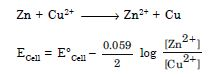ECell decreases when concentration of Zn2+ ions [Zn2+] increases.

##### Question 61:

What advantage do the fuel cells have over primary and secondary batteries?

Primary batteries contain a limited amount of reactants and are discharged when the reactants have been consumed. Secondary batteries can be recharged but take a long time to recharge. Fuel cell runs continuously as long as the reactants are supplied to it and products are removed continuously.

##### Question 62:

Write the cell reaction of a lead storage battery when it is discharged. How does the density of the electrolyte change when the battery is discharged?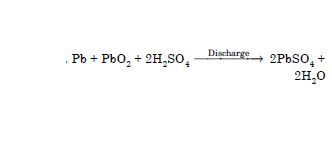Density of electrolyte decreases because water is formed and sulphuric acid is consumed as the product during discharge of the battery.

##### Question 63:

Why on dilution the $\mathrm{\Lambda }$m of CH3COOH increases drastically, while that of CH3COONa increases gradually?